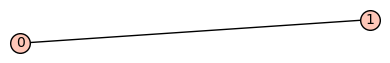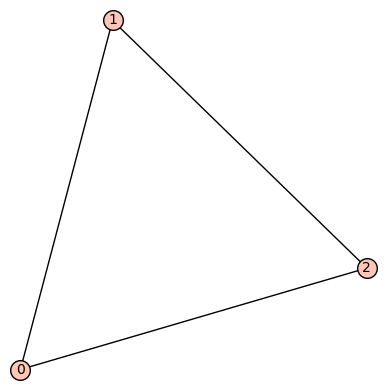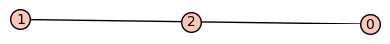# Obtaining all connected simply laced graphs with Sage for GAP

I work with GAP and have not much experience with Sage. I wonder whether there is an easy way to obtain a program with Sage that gives as output a list of all directed simply laced acyclic connected graphs on n points up to equivalence that is readable for GAP (via the GAP-package QPA). I know that Sage can generate the list of such combinatorial collections in an easy way but I do not know how to present it in the needed output and how to obtain all restrictions on the graphs. With acyclic I mean acyclic as an directed graph (but in case this makes problems, you can also assume acyclic as undirected graphs).

Here a more detailed description of what the program should do:

Input: A natural number n >= 2.

Output: The list of directed simply laced acyclic connected graphs on n points up to equivalence. Here we call two such graphs equivalent in case they have the same underlying undirected graph (in case it is a problem to do it up to equivalence, we can first look at the problem without up to equivalence). It is not important what the concrete orientation of the arrows of a representative in an equivalence class is.

Note that graphs are also often called quivers. GAP reads those graphs as Quiver(n, [[p1, p2, "a1"], ...,[pm, pn, "ar"]]) where we name the points pi and the arrows between them as aj for some index sets i and j. (I hope it will be clear with the following examples). Here how to output should look in the first 2 cases for n <= 3 so that it is readable for GAP:

n=2: [Quiver(2,[[1,2,"a1"]])];

n=3: [Quiver(3,[[1,2,"a1"],[2,3,"a2"]]),Quiver(3,[[1,2,"a1"],[2,3,"a2"],[1,3,"a3"]])]; (note for example here here that the quiver Quiver(3,[[1,2,"a1"],[2,3,"a2"]]) is equivalent to the quiver Quiver(3,[[1,2,"a1"],[3,2,"a2"]]) since they are equal when looking at their undirected graphs)

So for n=2 there is one equivalence class, while for n=3 there are two (so that for n=3 the output is a list of two elements readable by GAP).

edit retag close merge delete

Sort by » oldest newest most voted

My understanding is that, since you are looking for digraphs up to edge-orientation, and since every undirected graph can be oriented in an acyclic way (just define a linear order on the vertices the vertices and orient the edges accordingly), you are looking for connected undirected graphs up to isomorphism. Sage does that easily:

For example, imagine that you want to plot each of them, you can do:

sage: n = 2
sage: for G in graphs.nauty_geng('{} -c'.format(n)):
....:     show(G)


This will result as a single graph:If you set n=3, you will get:and so on.

Regarding your string representation, i am not sure how you plan to pass it to gap, but you can easily enumerate the edges of a graph G as follows:

sage: [[a,b,"a_{}".format(i)] for (i,(a,b)) in enumerate(G.edges(labels=False))]
[[0, 1, 'a_0'], [0, 2, 'a_1'], [1, 2, 'a_2']]

more

Thank you very much. In order for GAP to read it, the output should look as follows for example for n=3: [Quiver(3,[[1,2,"a1"],[2,3,"a2"]]),Quiver(3,[[1,2,"a1"],[2,3,"a2"],[1,3,"a3"]])]; So there are two problems. Namely SAGE enumeratres the vertices from 0 to n-1 instead of 1 to n (which is important for GAP). The other problem is that instead of "a1", SAGE uses 'a_1' (I think the underline after the a gives no problem but the ' instead of the " is a problem). Do you know an easy fix so that after writing n=3 at the beginning, the program gives the desired output? I tried some things but SAGE gives me some errors.

It would also be ok in case for n=3 (as an example), the output is just [ [[1,2,"a1"],[2,3,"a2"]] ,[ [1,2,"a1"],[2,3,"a2"],[1,3,"a3"]] ] (which is a list of two elements for GAP), since I can add the rest (Quiver(3,....) myself with GAP afterwards.